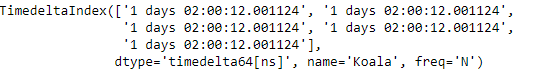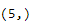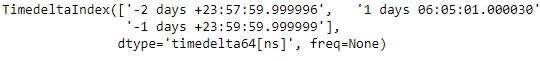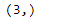# Python | Pandas TimedeltaIndex.shape

• Last Updated : 29 Dec, 2018

Python is a great language for doing data analysis, primarily because of the fantastic ecosystem of data-centric python packages. Pandas is one of those packages and makes importing and analyzing data much easier.

Pandas` TimedeltaIndex.shape` attribute return a tuple of the shape of the underlying data present in the object under consideration.

Syntax : TimedeltaIndex.shape

Return : shape

Example #1: Use `TimedeltaIndex.shape` attribute to find out the shape of the underlying data in the given object.

 `# importing pandas as pd``import` `pandas as pd`` ` `# Create the TimedeltaIndex object``tidx ``=` `pd.TimedeltaIndex(start ``=``'1 days 02:00:12.001124'``, periods ``=` `5``,``                                             ``freq ``=``'N'``, name ``=``'Koala'``)`` ` `# Print the TimedeltaIndex``print``(tidx)`

Output :Now we will find out the shape of the given object.

 `# print the shape of the tidx object``tidx.shape`

Output :As we can see in the output, the `TimedeltaIndex.shape` attribute has returned the (5, ) which is the shape of the underlying data of the tidx object.

Example #2: Use `TimedeltaIndex.shape` attribute to find out the shape of the underlying data in the given object.

 `# importing pandas as pd``import` `pandas as pd`` ` `# Create the TimedeltaIndex object``tidx ``=` `pd.TimedeltaIndex(data ``=``[``'-1 days 2 min 3us 10ns'``, ``'1 days 06:05:01.000030'``,``                                                      ``'-1 days + 23:59:59.999999'``])`` ` `# Print the TimedeltaIndex``print``(tidx)`

Output :Now we will find out the shape of the given object.

 `# print the shape of the tidx object``tidx.shape`

Output :As we can see in the output, the `TimedeltaIndex.shape` attribute has returned the (3, ) which is the shape of the underlying data of the tidx object.

My Personal Notes arrow_drop_up